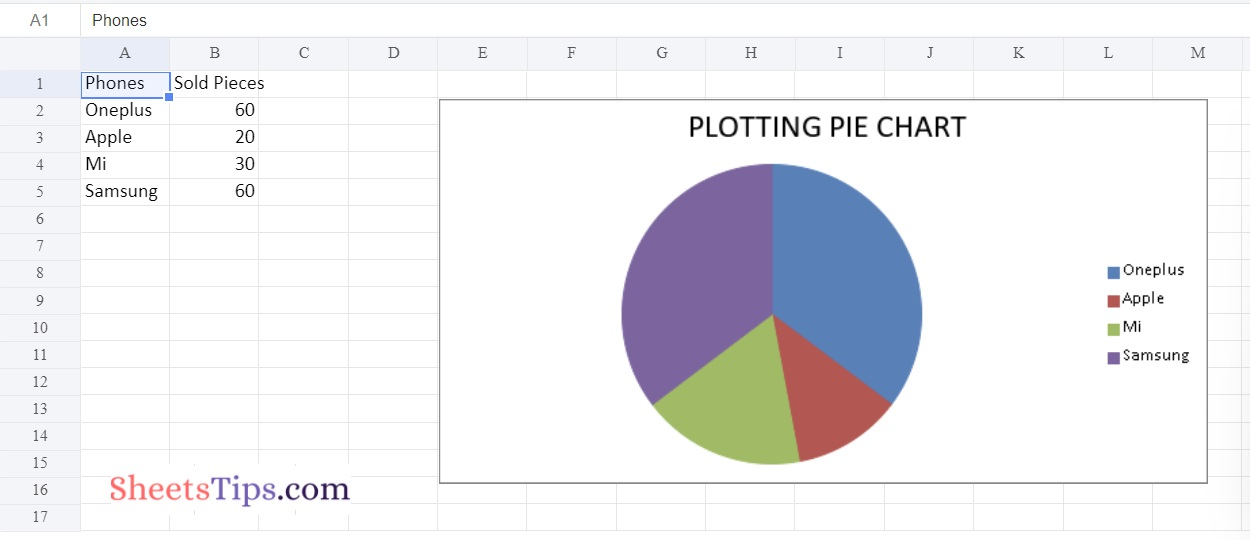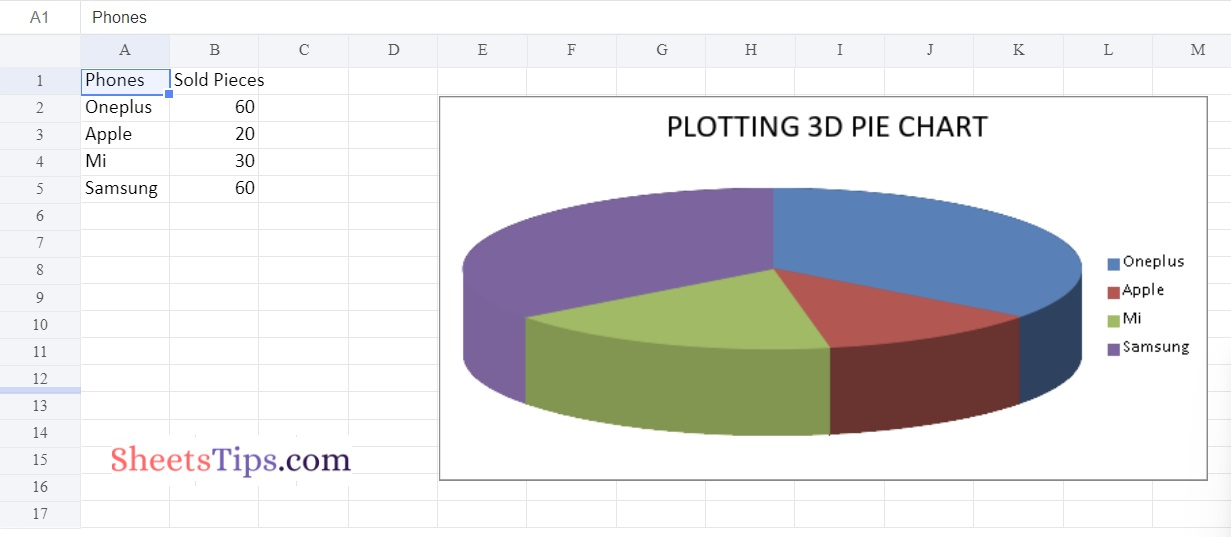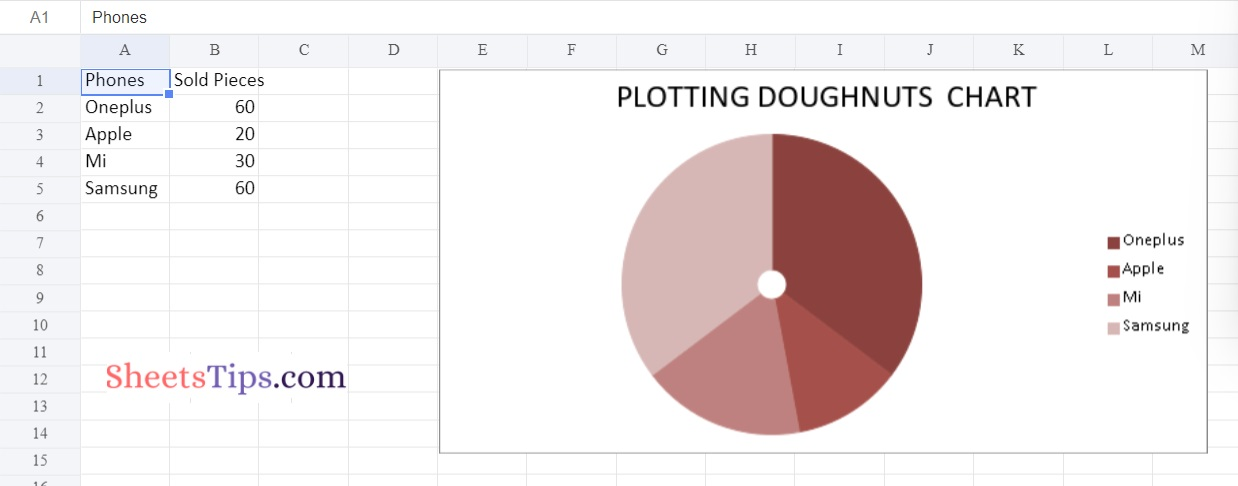# Python Program Plotting Charts in Excel Sheet using openpyxl module | Set 3

One of the most significant Python packages, Openpyxl, enables users to automate a variety of Excel tasks, including reading, writing, mathematical calculations, creating graphs, and more. Let’s talk about how to use Openpyxl to make charts on an Excel sheet without launching the Excel sheet in this article. Before plotting charts on Excel, we must first create a chart object of a specific class in Python in order to create a chart on an Excel sheet. After creating a chart object, we must populate it with data before putting it in the sheet object. The tutorial provides step-by-step instructions on how to do this in Python. Continue reading to learn more.

using openpyxl module in Python.

Openpyxl Module:

OpenPyXL is a Python package that allows you to communicate with Excel (.xlxs) files. But you can’t do it with Pandas. Yes, however Pandas does not support charts, formulas, or images. OpenPyXL allows data scientists and analysts to conduct a wide range of operations on Excel files, including:

• Creating and renaming sheets.
• Drawing/Plotting charts.
• Creating pivot tables.
• Inserting images, and even doing formatting and styling.

Another noteworthy feature of OpenPyXL is that it does not require the installation of Microsoft Excel.

## Program Plotting Charts in Excel Sheet using Openpyxl module in Python | Set 3

### Chart #1: Plotting the Pie Chart

Approach:

• Import the openpyxl module using the import keyword.
• Import the PieChart, Reference class from openpyxl.chart submodule using the import, from keywords.
• Create a workbook using the Workbook() function of the openpyxl module and store it in a variable.
• Set the above workbook to active by applying the active attribute to the workbook and store this sheet in a variable.
• Give the Data Values of the rows as a list and store them in a variable.
• Fill out the first, second, and third columns of the active sheet with the content of each row.
• Create the PieChart() object using the PieChart() function and store this in a variable.
• Creating Data to Plot.
• Add the data Values to the Pie chart Object using the add_data() function and set titles_from_data as True.
• Set the categories of the chart object by passing the label data as an argument to the set_categories() function.
• Set the title of the Chart Object using the title attribute.
• Add the above chartObject to the sheet using the add_chart() function by passing the chartObject and anchored cell values as arguments to it.
• Save the workbook by passing the fileName of the plot as an argument to the save() function.
• The Exit of the Program.

Below is the Implementation:

```# import the openpyxl module using the import keyword
import openpyxl

# import the PieChart,Reference class from openpyxl.chart sub module using the import,from keywords
from openpyxl.chart import PieChart,Reference

# Create a workbook using the Workbook() function of the openpyxl module and store it in a variable
workbook = openpyxl.Workbook()

# Set the above workbook to active by applying the active attribute to the workbook and store this sheet in a variable
worksheet = workbook.active

# Give the Data Values of the rows as a list and store it in a variable
rowsData = [
['Phones', 'Sold Pieces'],
['Oneplus', 60],
['Apple', 20],
['Mi', 30],
['Samsung', 60],
]

# Fill out the first, second, and third columns of the active sheet with the content of each row.
for rowVal in rowsData:
worksheet.append(rowVal)

# Create the PieChart() object using the PieChart() function and store this in a variable
chartObj = PieChart()

# Creating Data to Plot
labelData = Reference(worksheet, min_col = 1,
min_row = 2, max_row = 5)

dataValues = Reference(worksheet, min_col = 2,
min_row = 1, max_row = 5)

# Add the data Values to the Pie chart Object using the add_data() function and set titles_from_data as True

# Set the categories of the chart object by passing the labelData as argument to the set_categories() function
chartObj.set_categories(labelData)

# Set the title of the Chart Object using the title attribute
chartObj.title = " PLOTTING PIE CHART "

# Add the above chartObject to the sheet using the add_chart() function
# by passing the chartObject and anchored cell values as arguments to it.

# Save the workbook by passing the fileName of the plot as an argument to the save() function.
workbook.save("chartPie.xlsx")
```

Output:### Chart #2: Plotting 3D Pie Chart

Below is the Implementation:

```# import the openpyxl module using the import keyword
import openpyxl

# import the PieChart3D,Reference class from openpyxl.chart sub module using the import,from keywords
from openpyxl.chart import PieChart3D,Reference

# Create a workbook using the Workbook() function of the openpyxl module and store it in a variable
workbook = openpyxl.Workbook()

# Set the above workbook to active by applying the active attribute to the workbook and store this sheet in a variable
worksheet = workbook.active

# Give the Data Values of the rows as a list and store it in a variable
rowsData = [
['Phones', 'Sold Pieces'],
['Oneplus', 60],
['Apple', 20],
['Mi', 30],
['Samsung', 60],
]

# Fill out the first, second, and third columns of the active sheet with the content of each row.
for rowVal in rowsData:
worksheet.append(rowVal)

# Create the PieChart3D() object using the PieChart3D() function and store this in a variable
chartObj = PieChart3D()

# Creating Data to Plot
labelData = Reference(worksheet, min_col = 1,
min_row = 2, max_row = 5)

dataValues = Reference(worksheet, min_col = 2,
min_row = 1, max_row = 5)

# Add the data Values to the Pie chart Object using the add_data() function and set titles_from_data as True

# Set the categories of the chart object by passing the labelData as argument to the set_categories() function
chartObj.set_categories(labelData)

# Set the title of the Chart Object using the title attribute
chartObj.title = " PLOTTING 3D PIE CHART "

# Add the above chartObject to the sheet using the add_chart() function
# by passing the chartObject and anchored cell values as arguments to it.

# Save the workbook by passing the fileName of the plot as an argument to the save() function.
workbook.save("chartPie3D.xlsx")
```

Output:### Chart #3: Plotting Doughnut Chart

Below is the Implementation:

```# import the openpyxl module using the import keyword
import openpyxl

# import the DoughnutChart,Reference class from openpyxl.chart sub module using the import,from keywords
from openpyxl.chart import DoughnutChart,Reference

# import the DataPoint from openpyxl.chart.series class using the import keyword
from openpyxl.chart.series import DataPoint
# Create a workbook using the Workbook() function of the openpyxl module and store it in a variable
workbook = openpyxl.Workbook()

# Set the above workbook to active by applying the active attribute to the workbook and store this sheet in a variable
worksheet = workbook.active

# Give the Data Values of the rows as a list and store it in a variable
rowsData = [
['Phones', 'Sold Pieces'],
['Oneplus', 60],
['Apple', 20],
['Mi', 30],
['Samsung', 60],
]

# Fill out the first, second, and third columns of the active sheet with the content of each row.
for rowVal in rowsData:
worksheet.append(rowVal)

# Create the PieChart3D() object using the PieChart3D() function and store this in a variable
chartObj = DoughnutChart()

# Creating Data to Plot
labelData = Reference(worksheet, min_col = 1,
min_row = 2, max_row = 5)

dataValues = Reference(worksheet, min_col = 2,
min_row = 1, max_row = 5)

# Add the data Values to the Pie chart Object using the add_data() function and set titles_from_data as True

# Set the categories of the chart object by passing the labelData as argument to the set_categories() function
chartObj.set_categories(labelData)

# Set the title of the Chart Object using the title attribute
chartObj.title = " PLOTTING DOUGHNUTS  CHART "

#Set the style of the chart using the style attribute
chartObj.style = 28
# Add the above chartObject to the sheet using the add_chart() function
# by passing the chartObject and anchored cell values as arguments to it.